# Delta (Δ)

The sensitivity of a derivative's price to the underlying asset value.

Delta is a term used in trading to compare the change in the price of a derivative compared to the change in the price of the underlying asset. It is one of the 5 technical risk ratios and is sometimes known as the 'hedging ratio'.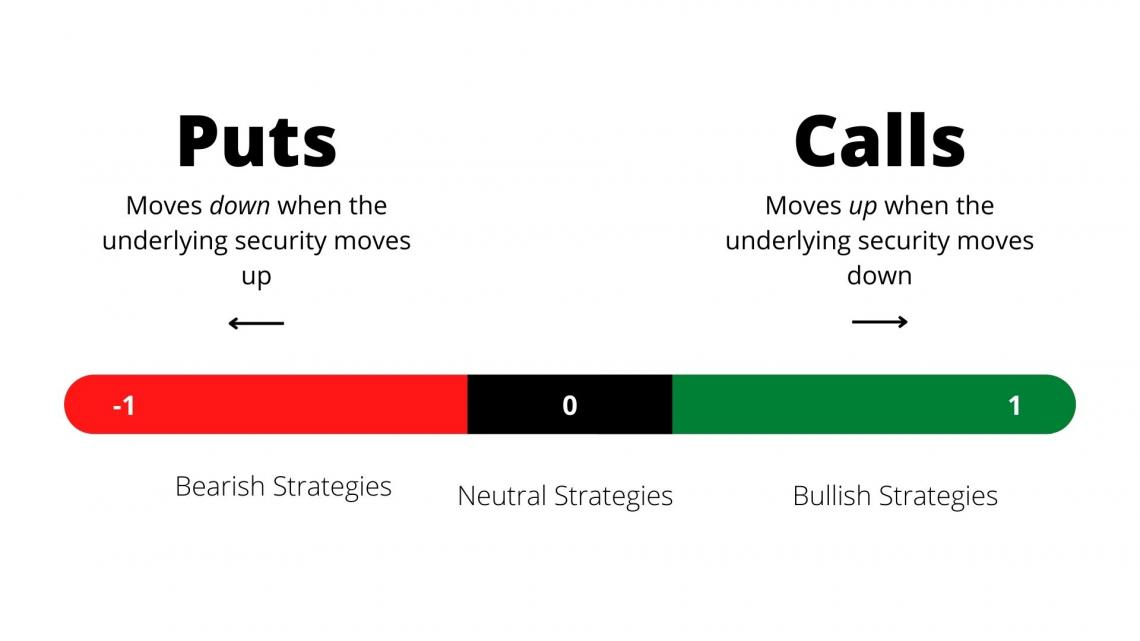It is used to calculate risk and whether a position is suitably hedged or not. An example of the delta is as follows:

• A call option on oil has a Δ of 1.2
• This means that for every \$1 that the price of oil increases, the value of the call option will increase by \$1.2

If an investor holds options as a means of hedging and they have a low delta, then the movement in option value will not offset any losses they experience on the underlying asset and therefore they are likely to be underhedged.

With options, as they near their expiry date their delta will tend to 1 (call) or -1 (put).

Delta (Δ) is a theoretical estimate of a rate of change in an option's value given a \$1 UP or DOWN move in the underlying security. The value of the Δ ranges from -1 to +1, where 0 represents an option, and the premium hardly moves relative to price changes in the stocks.

It is a positive value for long stocks, long calls, and short puts. Individually, long stock, long calls, and short puts are bullish strategies. Inversely,

Δ is a negative value for the short stock, short calls, and long puts. Conversely, short supply, short calls, and long puts are bearish strategies.

Δ is +1 for shares of long stock and -1 for shares of short supply. An option's Δ ranges from -1 to +1. The closer an option's Δ is to +1 or -1, the more strongly the option's premium responds to a change in the underlying security.

## How to Interpret Delta?

Delta is the ratio of change in the derivative price and the underlying asset price, and it can be positive or negative depending on the direction the derivative moves in relation to changes in the underlying asset.A call option will increase in value if the underlying asset increases in price; therefore, it has a positive Δ. Conversely, a put option will decrease in value if the underlying asset decreases in price; therefore, it has a negative Δ.

• For a put option, the value ranges from 0 to -1.
• For a call option, the value ranges from 0 to 1.

For example, a call option has a value of \$100, and the underlying asset has a price of \$115. The underlying asset increases in price to \$125, and the option value corresponds by increasing to \$105. The Δ is equal to: (\$105-\$100)/(\$125-\$115) = 0.5.

Another example is a put option with a value of \$100. The underlying asset has a price of \$150 and decreases to \$130. The corresponding put option value increases to \$107. The Δ here is equal to: (\$107-\$100)/(\$150-\$130) = -0.35Δ.

The Δ value will head away from 0 as an option moves further into the money/out of the money. A put option will head toward a value of -1, while a call option will head toward a value of 1.

Δ is -0.5 for a put option when it is at the money and 0.5 when a call option is at the money, meaning the strike price is equal to the underlying asset's price. It essentially says there is a 50/50 probability of the option ending up in or out of the money.

The delta sensitivity is affected by the time until the expiration of the option. The closer the option is to the expiration date, the more likely it will end up in its current state, whether in the money, out the money, or at the money. If a call option is in the money, the value will move closer to one as it approaches expiration.

## How is it used?

Δ indicates the option's price sensitivity to the underlying asset price. It can help to gauge the likelihood an option will expire in the money, meaning its strike price is below (for calls) or above (for puts) the underlying security's market price.Δ can also be seen as the probability of the option expiring in the money (ITM). This is why an at-the-money option has Δ of around 0.5 (50-50 chance), deep-in-the-money options have a Δ closer to 1, and deep-on-the-money options have a Δ closer to 0.

Hedging with the help of Δ reduces the risk of price movements in the underlying asset by offsetting long and short positions

Δ are further used for various other purposes in options trading like:

Assess Directional Risk

Bullish strategies have a positive, and bearish strategies have a negative value of the ratio. Stocks and each leg of an option strategy have their own Delta. The Delta of the contracts and securities can be combined to assess the directional risk of the plan as a whole.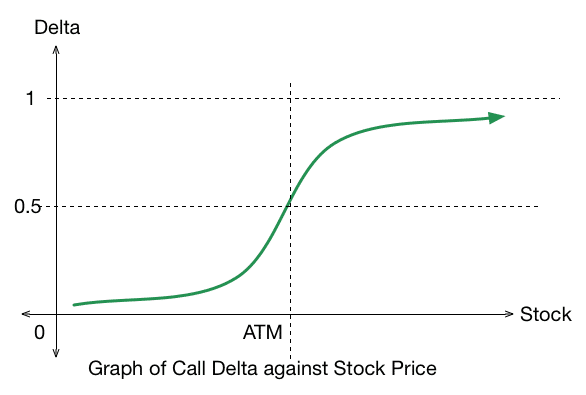Means - the net Deltas will reveal if a strategy or a portfolio is bullish or bearish.

For Example:

Long 100 XYZ equals +1 Δ (Long Stock, Bullish)

Short 1 XYZ call at -.30 Δ (Short Call, Bearish)

Net Δ = +.70 Δ

From a Δ perspective, this strategy is bullish, as demonstrated by a positive net Delta, and would benefit from upward movement of the underlying though to a lesser degree than the long stock position alone.

Assess Traction (Stock Sensitivity)

Short stocks have a Δ of -1, and Long stores have a Δ of +1. An option's Delta ranges from +1 to -1. The deeper an opportunity moves in the money, the closer an option's Delta moves toward +1 or -1.

Δ of +1 of an option will move in tandem with the underlying security, and it has begun acting like the stock. Meaning that the time value is no longer priced in, regardless of expiration.

Δ closer to +1 or -1 means a more remarkable change in the option price when the underlying moves.

Therefore, the sensitivity to the underlying security can be assessed individually by assessing Δ on an option contract or as a net figure from a strategy or portfolio perspective.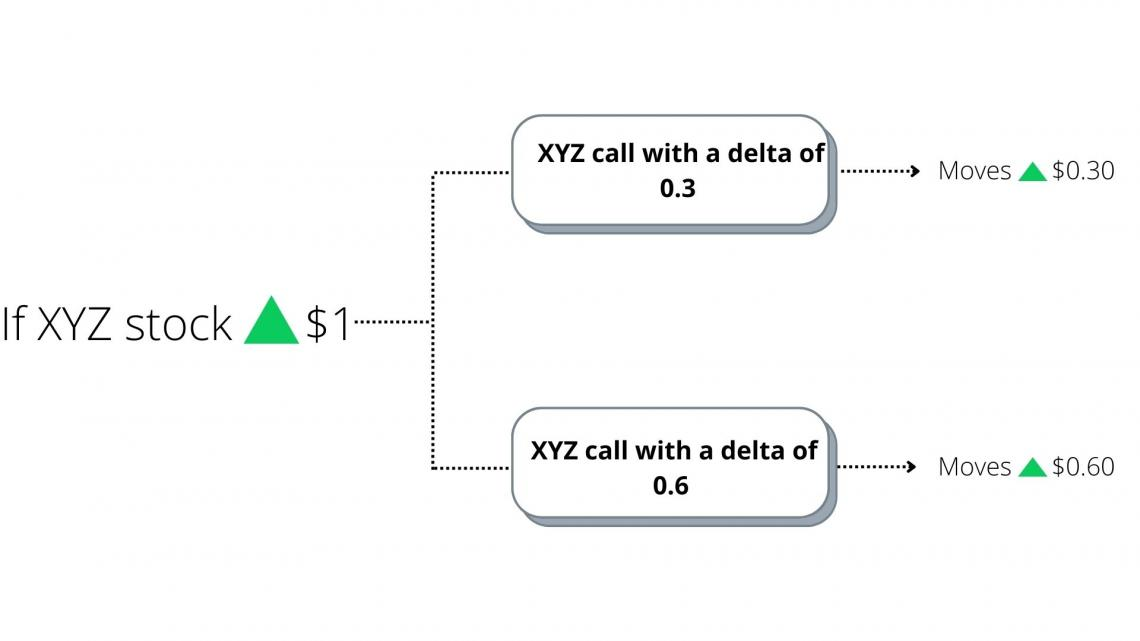Assess Probability of In-the-Money at Expiration

An increasing Δ indicates that the option is becoming more sensitive to the underlying security, and ultimately, the premium consists of primarily intrinsic value. It can be used in assessing the market-assigned probability of the option being in the money at expiration.

Δ closer to +1 or -1 indicates a more excellent intrinsic value, which can be translated into a higher probability of being in the money at expiration - potentially because it already is in the capital.

For example: In-the-money XYZ Call @ .60 Δ = 60% probability of being in-the-money at expiration

At-the-money XYZ Call @ .50 Δ = 50% probability of being in-the-money at expiration

Out-of-the-money XYZ Call @ .30 Δ = 30% probability of being in-the-money at expiration.

## Importance

It is one of the most important Greeks used for assessing derivatives. It can also be thought of as the probability that an option will end in the money.It is important to consider when determining the risk one is willing to take for a return on investment. It can also be used in assessing portfolios.

Looking at the aggregate portfolio can help determine how it would perform in relation to changes in the overall market.

Traders consider the sensitivity value as the amount of exposure to a stock or the underlying asset. The closer the value is to 1, the more exposed they are to the underlying asset.

The Δ value of an option can also be used to determine whether the options are being bought or sold.

If an option's price increases less than the Δ would imply, it could mean that traders are selling the option near the bid price. If the option's price is higher than the Δ implies, traders buy the options near the asking price.

## How can traders use them?

It is one of the most important Greeks used in assessing derivatives. It is crucial to consider when determining the risk one is willing to take for a return on investment.Observing the value in this way can be used in assessing portfolios. Looking at the aggregate portfolio can help determine how it would do with changes in the overall market.

Traders consider the sensitivity value as the amount of exposure to a stock or the underlying asset. When the value is closer to 1, they are more exposed to the underlying asset.

The Δ of an option can also be used to determine whether the options are being sold or bought.

If the price of an option is higher than the Δ would imply, it implies traders bought the options near the asking price.

If the price of an option is lower than the Δ would imply, it implies traders are selling the option near the bid price.

Also, It can be used for hedging purposes. The most common hedging strategy used is the neutral delta strategy which involves holding several options that, when taken in aggregate, are equal to or close to 0. it reduces the movement in options pricing relative to the underlying asset's price.

It can be used to tell

1. how much option contract's price will change based on a dollar move in the underlying

2. share equivalency, i.e., how many shares of the underlying your option contract are equivalent.

3. an approximation of the probability that the option contract will expire in/out of the money

Example: A long call with a 60 Δ; should move approximately \$0.60 with a \$1 move in the underlying (all else being equal). This is the equivalent of being long 60 shares of the underlying and has a 60% chance of being in/out of the money at expiration.

Now, how is it calculated?

Δ can be shown in its general form: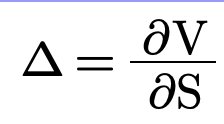Where:

• ∂ – the first derivative
• V – the option's price (theoretical value)
• S – the underlying asset's price

Under the Black-Scholes model, Δ is calculated by the following equation: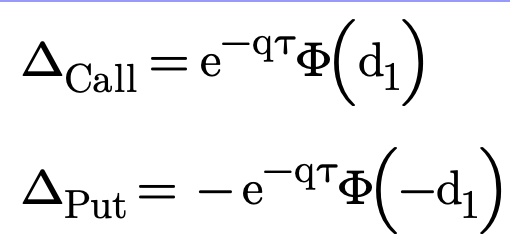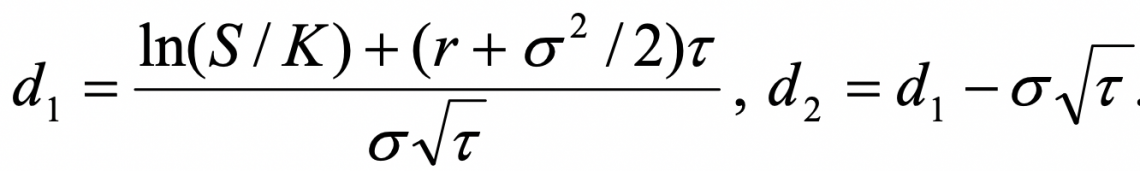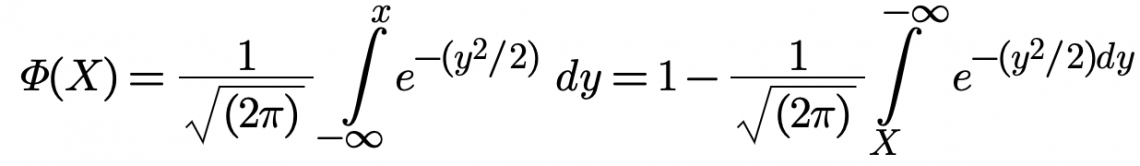Where:

• S – the stock price
• K – the strike price
• r – the risk-free rate
• q – the annual dividend yield
• τ – time until expiration
• σ – the volatility

## Examples

Let's assume a publicly-traded corporation called Oasis Inc. shares are traded on a stock exchange, and put-and-call options are traded for those shares.The Δ for the call option on Oasis Inc shares is 0.55. That means that a \$1 change in the price of Oasis Inc stock generates a \$0.55 difference in the price of Oasis Inc. call options.

Thus, if Oasis' shares trade at \$10 and the call option trades at \$1, a change in the price of Oasis shares to \$12 means the call option will increase to a price of \$1.55.

Put options work oppositely. If the put option on Oasis Inc. shares has a Δ of -\$0.45, then a \$1 increase in Oasis share price generates a \$.55 decrease in the price of Oasis put options. So if Oasis shares trade at \$10 and the put option trades at \$3, then Oasis shares increase to \$12, and the put option will decrease to a price of \$2.55.### Everything You Need To Master Excel Modeling

To Help You Thrive in the Most Prestigious Jobs on Wall Street.

Researched and authored by Rohan Kumar Singh | LinkedIn